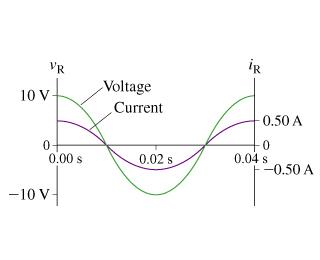# draw the resistor’s voltage and current phasors at t=15ms. [Physics help !!?]

edited

The figure (Figure 1) shows voltage and current graphs for a resistor.

I found part A , emf frequency = 25 Hz

Part B

What is the value of the resistance R?

Part C

Draw the resistor’s voltage and current phasors at t=15ms.selected by

R = V/i = 10/0.5 = 20Ω

V(t) = 10cos50πt = 10cos9000t where 9000t is in degrees because 50π rads/sec * 180°/π radians =9000

i(t) = 0.5cos50πt = 0.5cos9000t where 9000t is in degrees

At 15ms V = -7.07 and i = -0.3536A and they are exactly in phase with each other. 9000*15e-3 = 135°

Lorem ipsum dolor sit amet, consectetur adipiscing elit, sed do eiusmod tempor incididunt ut labore et dolore magna aliqua. Ut enim ad minim veniam, quis nostrud exercitation ullamco laboris nisi ut aliquip ex ea commodo consequat. Duis aute irure dolor in reprehenderit in voluptate velit esse cillum dolore eu fugiat.

+1 vote